## Number Visualization

math art kotlin

Simple algorithms to visualize numbers or sequences.

The general form of the algorithms is to walk a sequence of digits and move a cursor up, down, left, right, or diagonal. As the cursor moves it draws a pixel who's color is determined by other means.

Codebase can be found on GitHub

## π

### 100k digits, base 4, start from most significant bit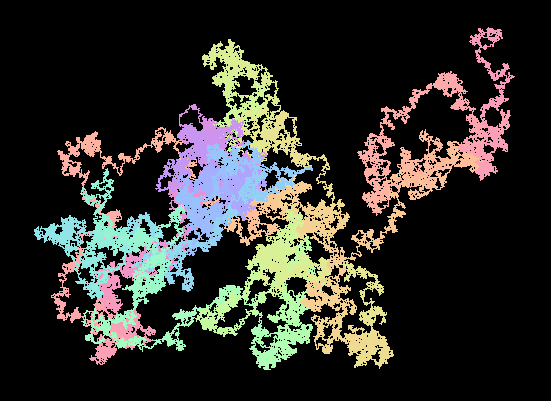### 100k digits, base 4, start from least significant bit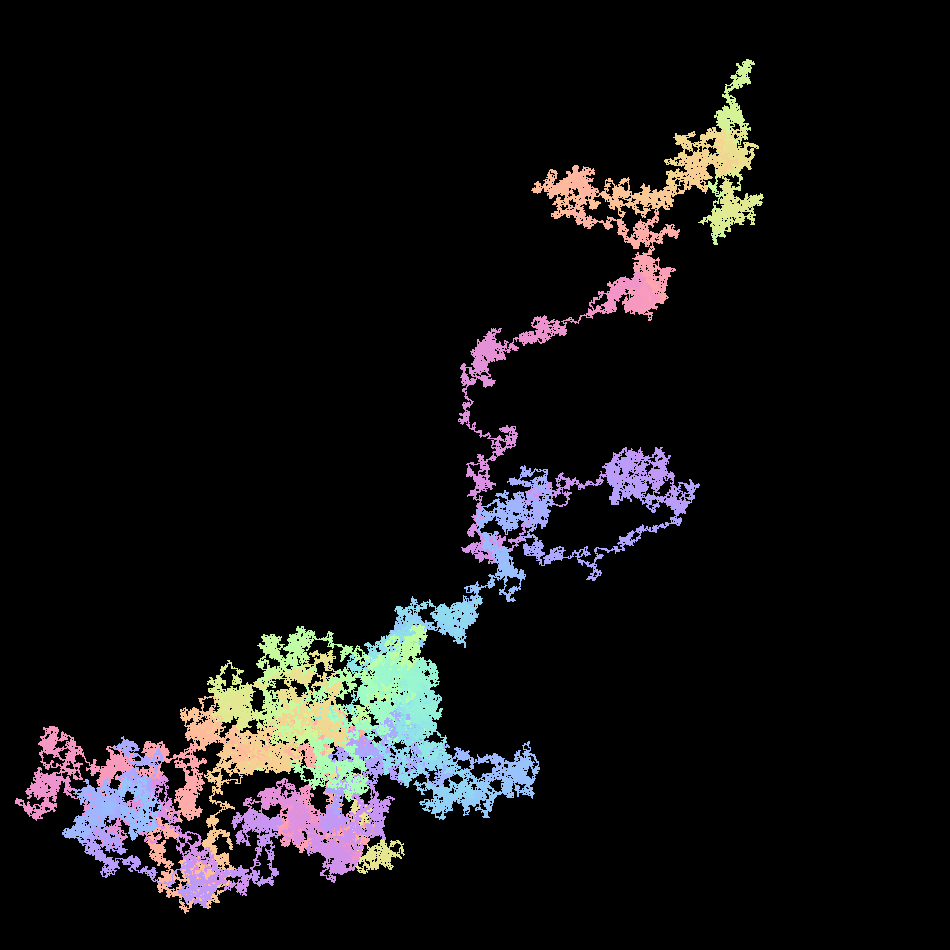## e

### 1,000,000 digits, base 4, start form most significant bit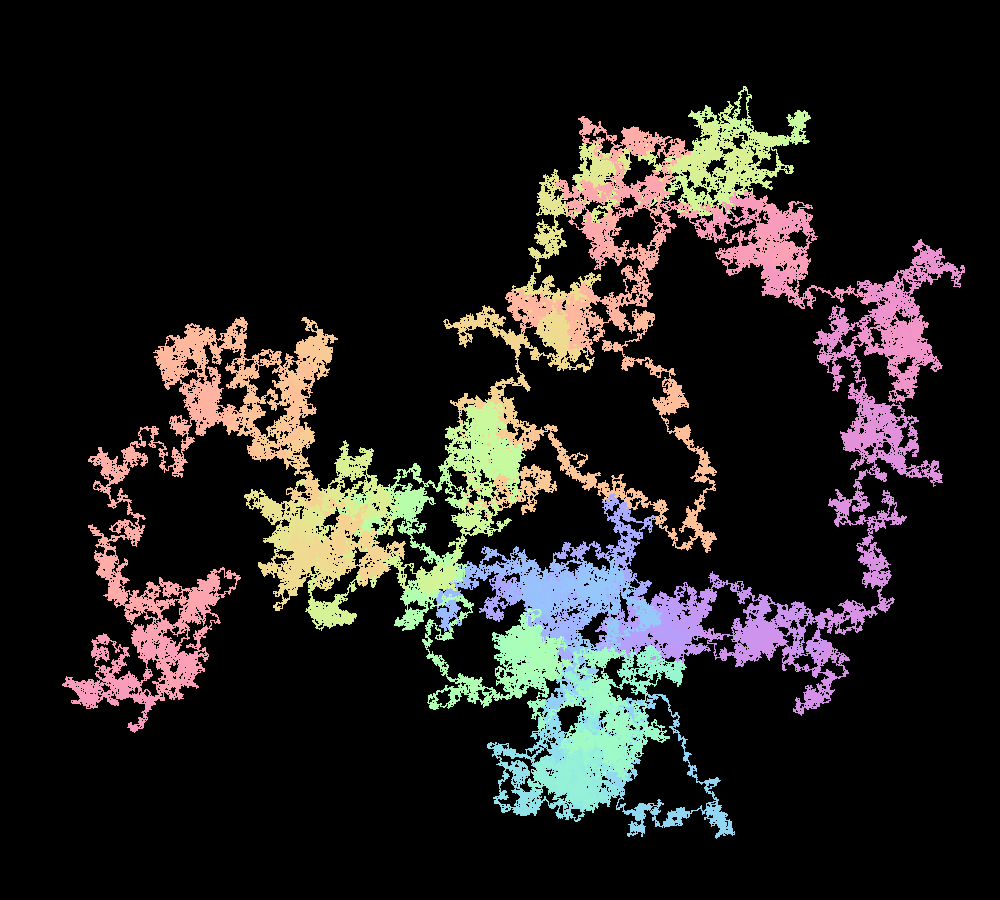### 1,000,000 digits, base 4, start from least significant bit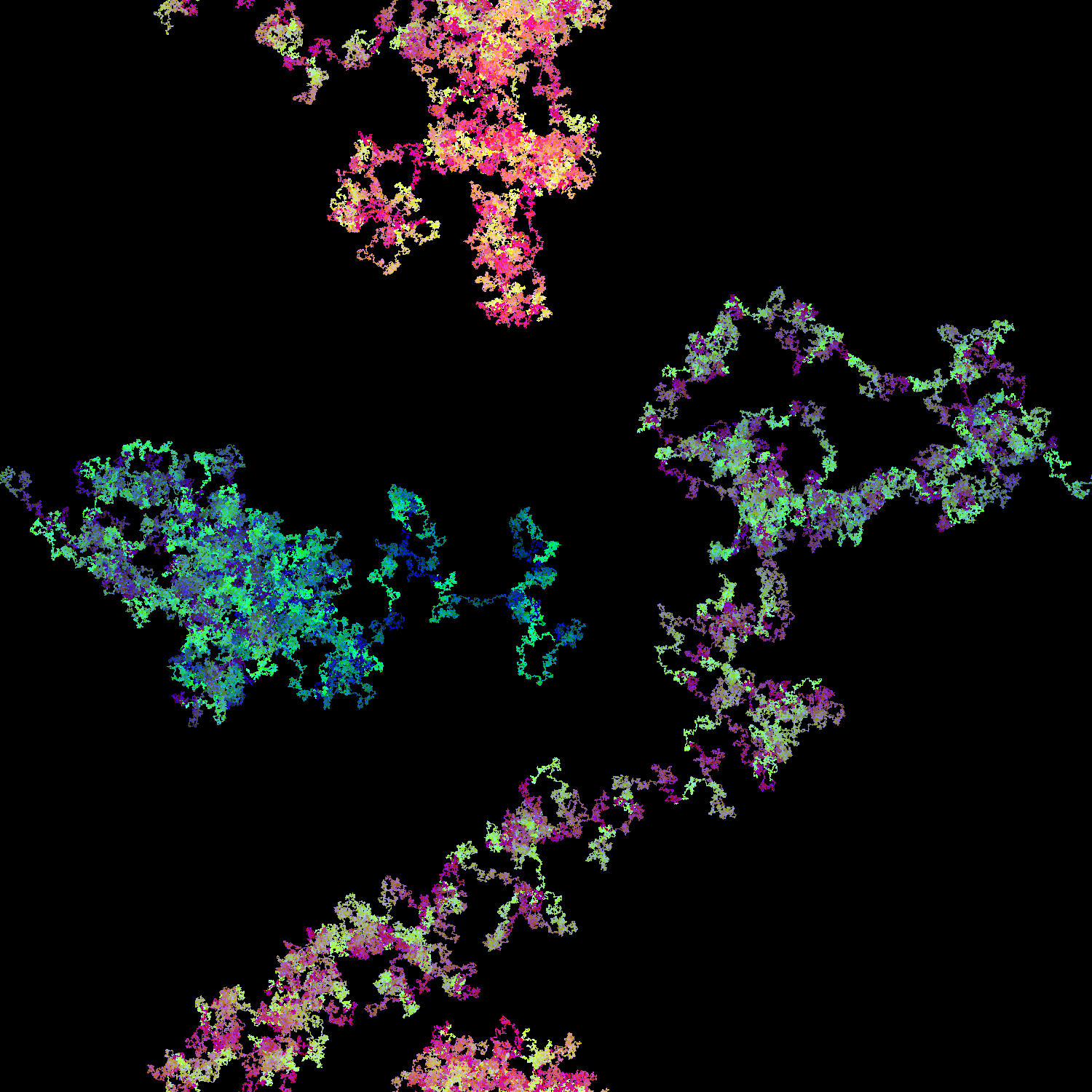### 1,000,000 digits, base 4, start from least significant bit, fill in space with decreasing color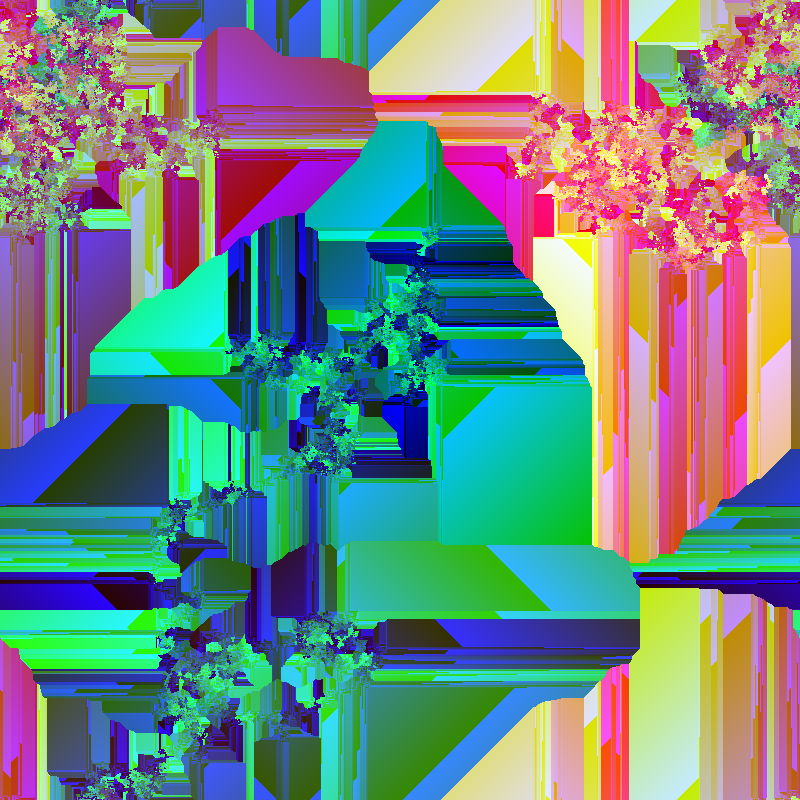## Mersenne 35 (2^1,398,269 - 1)

### base 10, 8 directions, start from most significant bit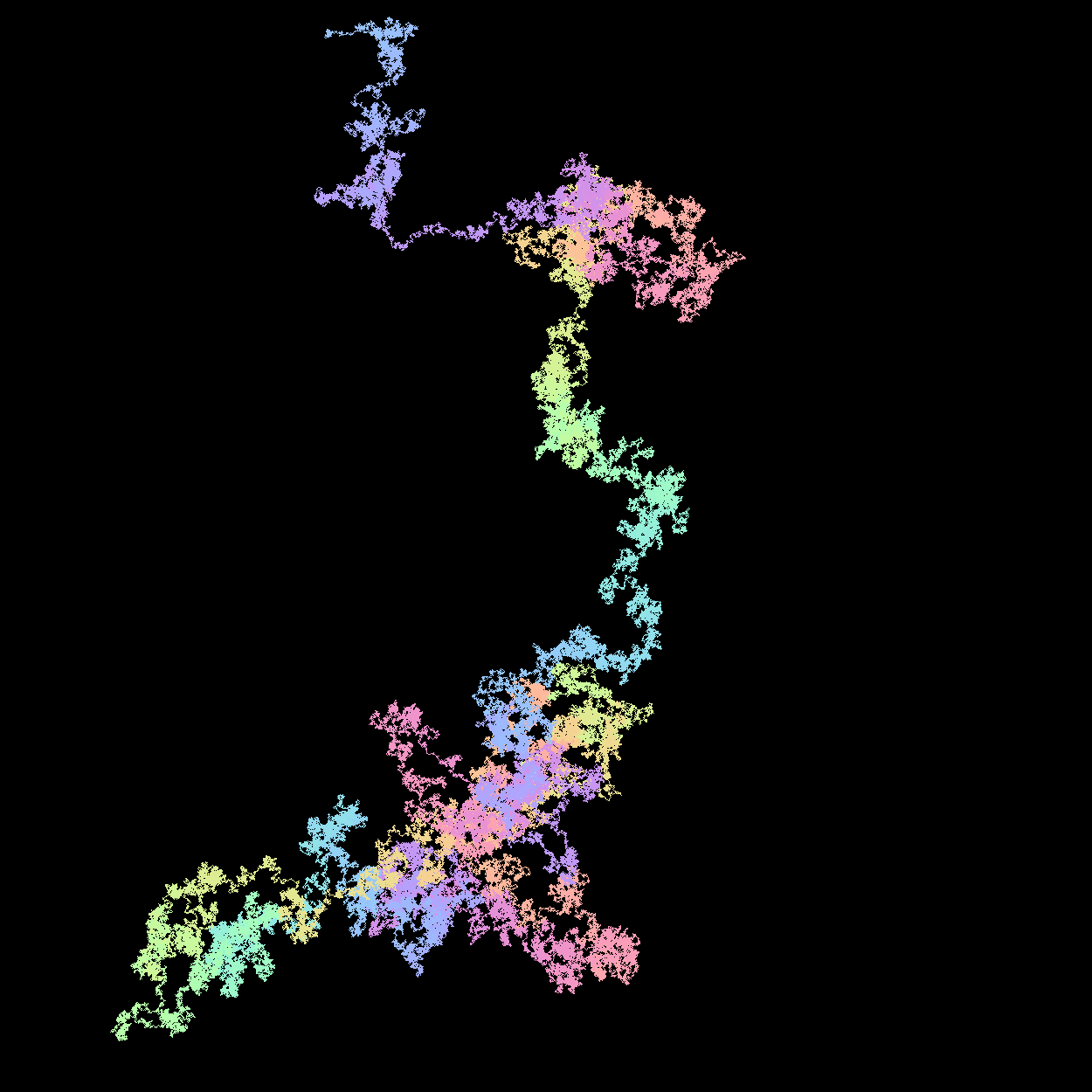## Misc Numbers & Sequences

### base 4, random digits (0-3)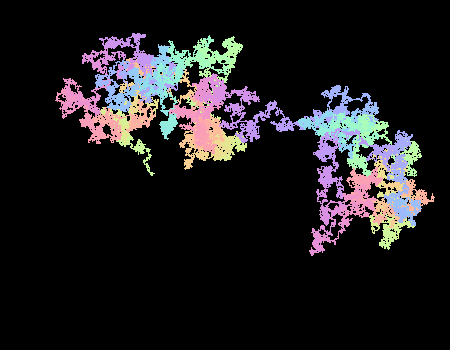To determine the color of the current pixel a value is stored in each (x,y) space equal to iteration/max_iteration * 0xFFFFFF.

The value can be mapped directly to an RGB. However, this does not result in a smooth color gradient. To generate a smooth gradient, I instead treat that value as an input into another function that "walks around" the color wheel (that you may see in many art apps). This results in a smoother color transition. More can be understood by reading this post. This post provides a very good starting point for understanding color gradients.

In code the color function is shown below. i in this case is the ith element in the sequence being plotted to the grid.

class SmoothColorizer(private val f1: Double = 0.3,
private val f2: Double = 0.3,
private val f3: Double = 0.3,
private val p1: Double = 0.0,
private val p2: Double = 2.0,
private val p3: Double = 4.0,
private val center: Int = 128,
private val width: Int = 127) : Colorizer {

override fun apply(i: Int): Color {
val r = Math.sin(f1 * i + p1) * width + center
val g = Math.sin(f2 * i + p2) * width + center
val b = Math.sin(f3 * i + p3) * width + center

return Color(r.toInt(), g.toInt(), b.toInt())
}
}